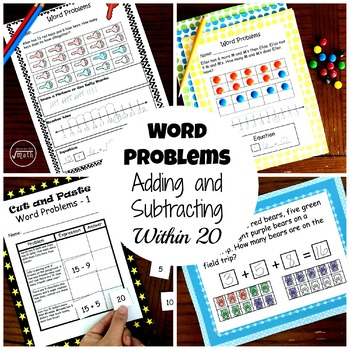# Adding and Subtracting Word Problems Within 20 - 4 Different ActivitiesResource Type
Format
PDF (2 MB|30 pages)
Standards
\$3.00
\$3.00

### Description

This Bundle contains four sets of word problems that involve adding and subtracting within 20.

M & M's Word Problem - This product has five adding and subtracting word problems that can be solved using M&M's (or with a q-tip and paint), a number line, and an equation.

Coloring Word Problems - This product has five adding and subtracting word problems that can be solved by coloring in objects in a ten frame, drawing or using tally marks, using a number line, and creating an equation.

Cut and Paste Word Problems - This product has four sheets with four-word problems on each sheet. Students find an expression and the answer that matches the word problem.

Teddy Bear Word Problems - This product has 20 adding and subtracting word problems within 20. Many of them have 3 addends.

CCSS.MATH.CONTENT.1.OA.A.1
Use addition and subtraction within 20 to solve word problems involving situations of adding to, taking from, putting together, taking apart, and comparing, with unknowns in all positions, e.g., by using objects, drawings, and equations with a symbol for the unknown number to represent the problem

CCSS.MATH.CONTENT.1.OA.A.2
Solve word problems that call for addition of three whole numbers whose sum is less than or equal to 20, e.g., by using objects, drawings, and equations with a symbol for the unknown number to represent the problem.
Total Pages
30 pages
N/A
Teaching Duration
1 Week
Report this Resource to TpT
Reported resources will be reviewed by our team. Report this resource to let us know if this resource violates TpT’s content guidelines.

### Standards

to see state-specific standards (only available in the US).
Solve word problems that call for addition of three whole numbers whose sum is less than or equal to 20, e.g., by using objects, drawings, and equations with a symbol for the unknown number to represent the problem.
Use addition and subtraction within 20 to solve word problems involving situations of adding to, taking from, putting together, taking apart, and comparing, with unknowns in all positions, e.g., by using objects, drawings, and equations with a symbol for the unknown number to represent the problem.